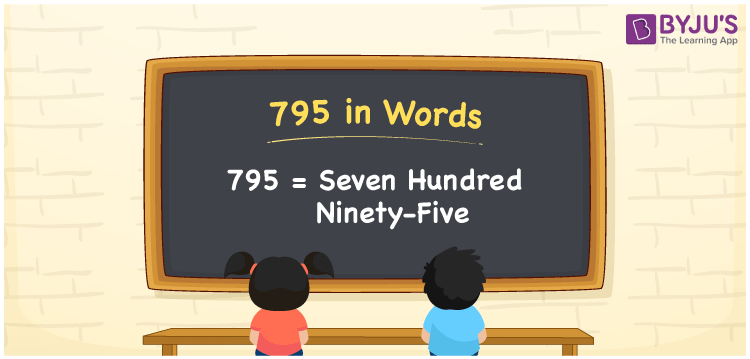# 795 in Words

The number 795 has three digits and it is spelt as “Seven hundred ninety-five”. In Mathematics, 795 is also considered to be a cardinal number. For example, the cost of 20 icecreams is Rs. 795. For writing the number 795 in words, the place value system plays an important role. Now, let us discuss the spelling of 795 in English words in this article.

 795 in Words: Seven Hundred Ninety-five. Seven Hundred Ninety-five in Numerical Form: 795.

## 795 in English Words## How to Write 795 in Words?

The number 795 has three digits and its place value table is given below:

 Hundreds Tens Ones 7 9 5

The expanded form of 795 is as follows:

= 7 × Hundred + 9 × Ten + 5 × One

= 7 × 100 + 9 × 10 + 5 × 1

= 700 + 90 + 5

= 795

= Seven hundred ninety-five

Hence, 795 in words is seven hundred ninety-five.

795 in words – Seven hundred ninety-five

Is 795 an odd number? – Yes

Is 795 an even number? – No

Is 795 a perfect square number? – No

Is 795 a perfect cube number? – No

Is 795 a prime number? – No

Is 795 a composite number? – Yes

## Frequently Asked Questions on 795 in Words

Q1

### How to spell 795 in words?

795 in words is seven hundred ninety-five.

Q2

### Simplify 700 + 95, and express it in words.

Simplifying 700 + 95, we get 795. Hence, 795 in words is seven hundred ninety-five.

Q3

### Is 795 an even number?

No, 795 is not an even number.